Author: Oscar Cronquist Article last updated on September 13, 2021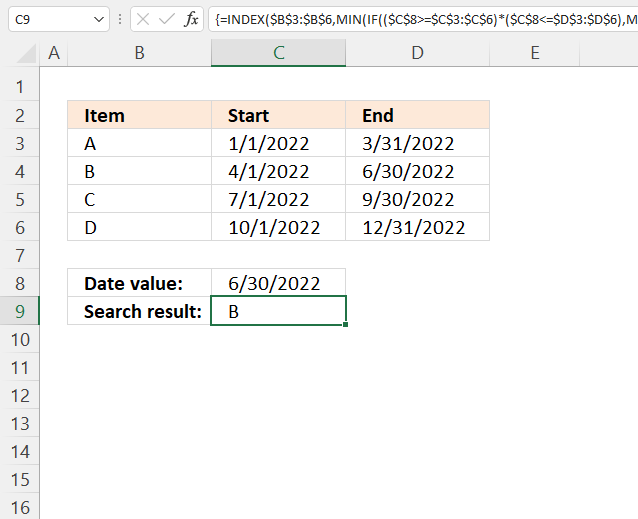This article demonstrates how to match a specified date to date ranges. The image above shows a formula in cell C9 that uses the date specified in cell C8 to match a date range.

A date range is matched if the date is larger or equal to the start date and smaller or equal to the end date. The date ranges are in cell range C3:D6.

The date in cell C8 matches date range 4-1-2022 / 6-30-2022, the formula returns a value on the same row from cell range B3:B6, in this case, Item "B".

## 1. Match a date when a date range is entered in a single cell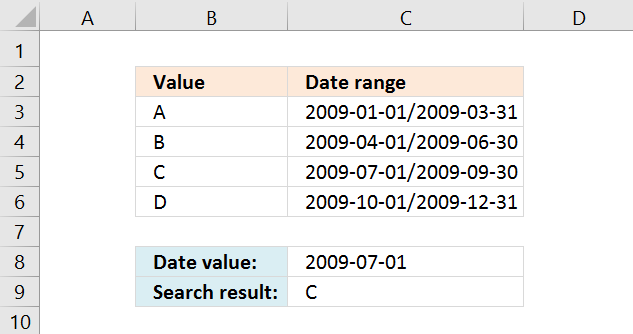Column C contains the start and end date separated by a forward slash /. The formula in cell C9 splits the dates and checks if the date in cell C8 is in a date range and if it is it returns the adjacent value on the same row.

Formula in C9:

=INDEX(B3:B6, SUMPRODUCT((DATEVALUE(LEFT(C3:C6, FIND("/", C3:C6)-1))<=C8)*(DATEVALUE(RIGHT(C3:C6, LEN(C3:C6)-FIND("/", C3:C6)))>=C8), ROW(C3:C6)-MIN(ROW(C3:C6))+1))

### 1.2 How to create an array formula

1. Select cell C9.
2. Press with left mouse button on in the formula bar.
3. Copy and paste array formula to the formula bar.
4. Press and hold CTRL + SHIFT simultaneously.
5. Press Enter.
6. Release all keys.

### 1.3 Explaining formula

#### Step 1 - Calculate character position of forward slash

The FIND function returns the position of a specific string in another string, reading left to right. Note, the FIND function is case-sensitive.

FIND(find_text,within_text, [start_num])

FIND("/", C3:C6)

becomes

FIND("/", {"2009-01-01/2009-03-31"; "2009-04-01/2009-06-30"; "2009-07-01/2009-09-30"; "2009-10-01/2009-12-31"})

and returns {11; 11; 11; 11}.

#### Step 2 - Calculate the number of characters in each cell

The LEN function returns the number of characters in a cell value.

LEN(text)

LEN(C3:C6)

becomes

LEN({"2009-01-01/2009-03-31"; "2009-04-01/2009-06-30"; "2009-07-01/2009-09-30"; "2009-10-01/2009-12-31"})

and returns {21; 21; 21; 21}.

#### Step 3 - Calculate position from last character

The minus character lets you perform an arithmetic operation, it subtracts one value with another value.

LEN(C3:C6)-FIND("/", C3:C6)

becomes

{21; 21; 21; 21} - {11; 11; 11; 11}

and returns {10; 10; 10; 10}.

#### Step 4 - Extract characters

The RIGHT function extracts a specific number of characters always starting from the right.

RIGHT(text,[num_chars])

RIGHT(C3:C6, LEN(C3:C6)-FIND("/", C3:C6))

becomes

RIGHT(C3:C6, {10; 10; 10; 10})

becomes

RIGHT({"01-01-2009/03-31-2009"; "04-01-2009/06-30-2009"; "07-01-2009/09-30-2009"; "10-01-2009/12-31-2009"}, {10; 10; 10; 10})

and returns

{"03-31-2009"; "06-30-2009"; "09-30-2009"; "12-31-2009"}

#### Step 5 - Convert text to date

The DATEVALUE function returns an Excel date value (serial number) based on a date stored as text.

DATEVALUE(date_text)

DATEVALUE(RIGHT(C3:C6, LEN(C3:C6)-FIND("/", C3:C6)))

becomes

DATEVALUE({"03-31-2009"; "06-30-2009"; "09-30-2009"; "12-31-2009"})

and returns {39903; 39994; 40086; 40178}.

#### Step 6 - Check if larger than or equal to

The larger than and equal signs are logical operators, they are used in this example to check if a date is in a date range.

The output is a boolean value TRUE or FALSE, the position of each value in the array correspond to the date ranges.

DATEVALUE(RIGHT(C3:C6, LEN(C3:C6)-FIND("/", C3:C6)))>=C8

becomes

{39814; 39904; 39995; 40087}<=39995

and returns

{TRUE; TRUE; TRUE; FALSE}.

#### Step 7 - Check date against end dates

DATEVALUE(RIGHT(C3:C6, LEN(C3:C6)-FIND("/", C3:C6)))>=C8

becomes

{39903; 39994; 40086; 40178}>=39995

and returns

{FALSE; FALSE; TRUE; TRUE}.

#### Step 8 - Create number sequence

The ROW function calculates the row number of a cell reference.

ROW(reference)

ROW(\$C\$3:\$C\$6)

returns

{3; 4; 5; 6}.

#### Step 9 - Create a number sequence from 1 to n

The MATCH function returns the relative position of an item in an array or cell reference that matches a specified value in a specific order.

MATCH(lookup_value, lookup_array, [match_type])

MATCH(ROW(\$C\$3:\$C\$6), ROW(\$C\$3:\$C\$6))

becomes

MATCH({3; 4; 5; 6}, {3; 4; 5; 6})

and returns

{1; 2; 3; 4}.

#### Step 10 - Multiply arrays

The asterisk charcater lets you multiply arrays.

(DATEVALUE(LEFT(C3:C6, FIND("/", C3:C6)-1))<=C8)*(DATEVALUE(RIGHT(C3:C6, LEN(C3:C6)-FIND("/", C3:C6)))>=C8), ROW(C3:C6)-MIN(ROW(C3:C6))+1)

becomes

{TRUE; TRUE; TRUE; FALSE} * {FALSE; FALSE; TRUE; TRUE} * {1; 2; 3; 4}

becomes

{0; 0; 1; 0} * {1; 2; 3; 4}

and returns

{0; 0; 3; 0}.

#### Step 11 - Sum numbers

The SUMPRODUCT function calculates the product of corresponding values and then returns the sum of each multiplication.

SUMPRODUCT((DATEVALUE(LEFT(C3:C6, FIND("/", C3:C6)-1))<=C8)*(DATEVALUE(RIGHT(C3:C6, LEN(C3:C6)-FIND("/", C3:C6)))>=C8), ROW(C3:C6)-MIN(ROW(C3:C6))+1))

becomes

SUMPRODUCT({0; 0; 3; 0})

and returns 3.

#### Step 12 - Get value

The INDEX function returns a value from a cell range, you specify which value based on a row and column number.

INDEX(array[row_num][column_num])

INDEX(\$B\$3:\$B\$6, SUMPRODUCT(DATEVALUE(RIGHT(C3:C6, LEN(C3:C6)-FIND("/", C3:C6)))>=C8)*(DATEVALUE(LEFT(C3:C6, FIND("/", C3:C6)-1))<=C8), MATCH(ROW(\$C\$3:\$C\$6), ROW(\$C\$3:\$C\$6))))

becomes

INDEX(\$B\$3:\$B\$6, 3)

and returns "C" in cell C9.### 1.4 Exel file, see sheet Ex 1

matching-a-date-in-a-date-range v3.xlsx
(Excel 2007- Workbook *.xlsx)

## 2. Match a date when a date range is entered in two cells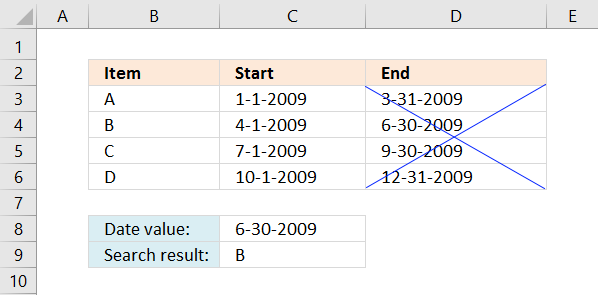There are no gaps between these date ranges which makes it is possible to only use the dates specified in column C in the following formula:

Formula in cell C9:

=LOOKUP(C8,C3:C6,B3:B6)

Note, there are no gaps between date ranges. If you have gaps use the formula in section 3.

### 2.2 Explaining formula

#### Step 1 - LOOKUP function

The LOOKUP function lets you find a value in a cell range and return a corresponding value on the same row.

LOOKUP(lookup_valuelookup_vector, [result_vector])

The values in lookup_vector must be sorted in ascending order or from A to Z.

#### Step 2 - Populate argumentsLOOKUP(C8,C3:C6,B3:B6)

lookup_value - The lookup value is in cell C9.

lookup_vector - The lookup cell range is C3:C6. Note start dates are sorted in ascending order.

[result_vector- The function returns a value from this range on the same row as the matching lookup value.

#### Step 3 - Evaluate LOOKUP function

LOOKUP(C8,C3:C6,B3:B6)

becomes

LOOKUP(39994, {39814; 39904; 39995; 40087}, {"A"; "B"; "C"; "D"})

and returns "B".## 3. Match a date when a date range is entered in two cells with gaps not sorted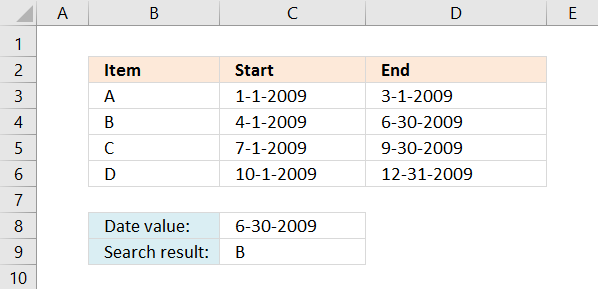Remember, the LOOKUP function requires date values to be sorted in an ascending order in column C. If not, use the formula below.

If you do have gaps between some date ranges, like the picture below. There is a date gap between 3-1-2009 and 4-1-2009. You then need to use both the start and end date to find the value you are looking for.

Formula in cell C9:

=INDEX(\$B\$3:\$B\$6,SUMPRODUCT((\$C\$8>=\$C\$3:\$C\$6)*(\$C\$8<=\$D\$3:\$D\$6)*MATCH(ROW(\$B\$3:\$B\$6),ROW(\$B\$3:\$B\$6))))

If the user enters a value outside the date ranges error #N/A is returned.

### 3.2 Explaining formula in cell C9

#### Step 1 - Check if the end dates is larger or equal to date

The less than, larger than, and equal signs are all logical operators. They return a boolean value True or False.

\$C\$8>=\$C\$3:\$C\$6

becomes

39994>={39814; 39904; 39995; 40087}

and returns

{TRUE; TRUE; FALSE; FALSE}.

#### Step 2 - Check if the start dates is smaller or equal to date

\$C\$8<=\$D\$3:\$D\$6

becomes

39994<={39903; 39994; 40086; 40178}

and returns

{FALSE; TRUE; TRUE; TRUE}.

#### Step 3 - Multiply arrays - AND logic

The parentheses let you control the order of calculation. The asterisk multiples two numbers or two arrays.

(\$C\$8>=\$C\$3:\$C\$6)*(\$C\$8<=\$D\$3:\$D\$6)

becomes

(39994>={39814; 39904; 39995; 40087})*(39994<={39903; 39994; 40086; 40178})

becomes

{TRUE; TRUE; FALSE; FALSE}*{FALSE; TRUE; TRUE; TRUE}

and returns

{0; 1; 0; 0}

#### Step 4 - Create a number sequence

The ROW function calculates the row number of a cell reference.

ROW(reference)

ROW(\$B\$3:\$B\$6)

returns {3; 4; 5; 6}.

#### Step 5 - Create a number sequence from 1 to n

The MATCH function returns the relative position of an item in an array or cell reference that matches a specified value in a specific order.

MATCH(lookup_value, lookup_array, [match_type])

MATCH(ROW(\$B\$3:\$B\$6), ROW(\$B\$3:\$B\$6))

becomes

MATCH({3; 4; 5; 6}, {3; 4; 5; 6})

and returns {1; 2; 3; 4}.

#### Step 6 - Multiply with relative row numbers

(\$C\$8>=\$C\$3:\$C\$6)*(\$C\$8<=\$D\$3:\$D\$6)* MATCH(ROW(\$B\$3:\$B\$6), ROW(\$B\$3:\$B\$6))

becomes

{0; 1; 0; 0}*MATCH(ROW(\$B\$3:\$B\$6), ROW(\$B\$3:\$B\$6))

becomes

{0; 1; 0; 0}*{1; 2; 3; 4}

and returns {0; 2; 0; 0}.

#### Step 7 - Sum values in the array

The SUMPRODUCT function calculates the product of corresponding values and then returns the sum of each multiplication. It also has the advantage that you don't need to enter the formula as an array formula.

SUMPRODUCT((\$C\$8>=\$C\$3:\$C\$6)*(\$C\$8<=\$D\$3:\$D\$6)* MATCH(ROW(\$B\$3:\$B\$6), ROW(\$B\$3:\$B\$6)))

becomes

SUMPRODUCT({0; 2; 0; 0})

and returns 2.

#### Step 8 - Return value from cell range

The INDEX function returns a value from a cell range, you specify which value based on a row and column number.

INDEX(array[row_num][column_num])

INDEX(\$B\$3:\$B\$6, SUMPRODUCT((\$C\$8>=\$C\$3:\$C\$6)*(\$C\$8<=\$D\$3:\$D\$6)* MATCH(ROW(\$B\$3:\$B\$6), ROW(\$B\$3:\$B\$6))))

becomes

INDEX(\$B\$3:\$B\$6, 2)

becomes

INDEX({"A"; "B"; "C"; "D"}, 2)

and returns "B" in cell C9. Value "B" is the second value in the array.

### Get the Excel filematching-a-date-in-a-date-range-v3.xlsx

## 4. Use VLOOKUP to search date in date ranges and return value on the same row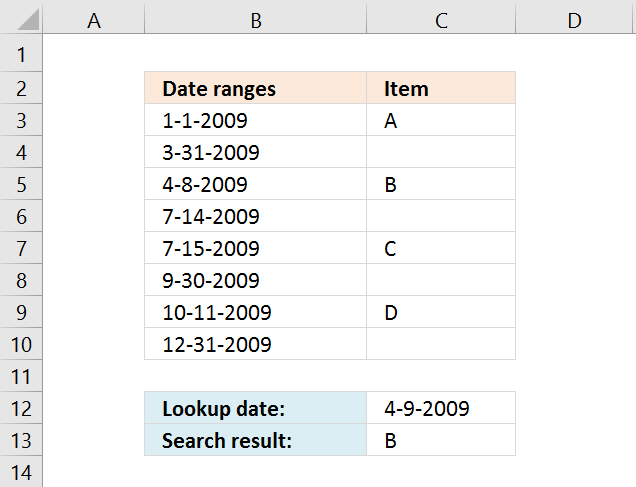The following formula uses only the VLOOKUP function, however, the dates must be sorted in ascending order and if a date is outside a date ranges 0 (zero) is returned. There can't be any overlapping date ranges and the formula can only return one value.

The example demonstrated in the image above has date ranges in only one column. Every other date is the end date of each date range.

You are also required to have the lookup column in the first column in the cell reference you use in the VLOOKUP function. Example, the second argument in the VLOOKUP function below is this cell reference: B3:C10. The lookup column must be in column B.

Formula in cell C13:

=VLOOKUP(C12,B3:C10,2,TRUE)

Important! The first date range seems to be 1-1-2009/3-31-2009 but it is actually 1-1-2009/3-30-2009, why is this? The VLOOKUP function matches the largest date that is smaller or equal to the lookup date. If the lookup date is 3-31-2009 it will match 3-31-2009 found in cell B4 and return the corresponding value in column C (cell C4). In this case nothing, cell C4 is empty. This applies to all date ranges in column B.

You need to change your date ranges accordingly if you want to use the VLOOKUP function for date ranges entered vertically. However, the VLOOKUP function works perfectly fine if you have date ranges with no gaps between the end dates and start dates, see picture below. You then only need to use the start dates for each date range, example demonstrated in column C see picture below.Recommended article

Recommended articles

How to return a value if lookup value is in a range
In this article, I will demonstrate four different formulas that allow you to lookup a value that is to be found […]

### 4.1 Explaining formula

VLOOKUP(C12,B3:C10,2,TRUE)

#### Step 1 - VLOOKUP function

The VLOOKUP function lets you search the leftmost column for a value and return another value on the same row in a column you specify.

VLOOKUP(lookup_value, table_array, col_index_num, [range_lookup])

The values in lookup_vector must be sorted in ascending order or from smallest to largest

#### Step 2 - Populate argumentsVLOOKUP(C12, B3:C10, 2, TRUE)

lookup_value - Date value specified in cell C12

table_array - A cell range containing both the lookup column and the return value column,in this case, B3:C10.

col_index_num - From which column to return a value from.

[range_lookup] - True or False (boolean value). True - approximate match, the leftmost column must be sorted in ascending order, or from small to large. False - Exact match.

#### Step 3 - Evaluate VLOOKUP function

VLOOKUP(C12, B3:C10, 2, TRUE)

becomes

VLOOKUP(39912, {39814, "A"; 39903, 0; 39911, "B"; 40008, 0; 40009, "C"; 40086, 0; 40097, "D"; 40178,0}, 2, TRUE)

and returns "B" in cell C13.

### 4.2 Get Excel file, see sheet Ex 4

matching-a-date-in-a-date-range v3.xlsx
(Excel 2007- Workbook *.xlsx)

## 5. Match a date when date ranges sometimes overlap and return multiple results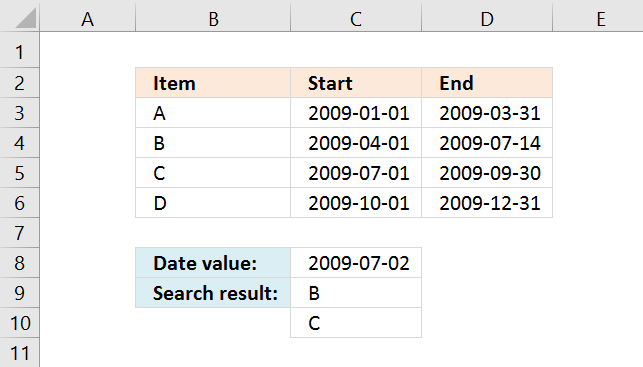This example demonstrates a formula that returns multiple values if a date condition is met in multiple date ranges. This is only possible if the date ranges overlap.

For example, date 7/2/2009 above matches both date ranges 4/1/2009 - 14/7/2009 and 7/1/2009 - 9/30/2009. The corresponding values to those date ranges are "B" and "C".

Array formula in cell C9:

=INDEX(\$B\$3:\$B\$6, SMALL(IF((\$C\$8>=\$C\$3:\$C\$6)*(\$C\$8<=\$D\$3:\$D\$6), MATCH(ROW(\$B\$3:\$B\$6), ROW(\$B\$3:\$B\$6))), ROW(A1)))

How to enter an array formula

#### How to copy an array formula

1. Select cell C9.
2. Copy cell (not formula). (shortcut keys CTRL + c)
3. Select cell range C10:C11.
4. Paste (shortcut keys CTRL + v).

### 5.1 Explaining formula

#### Step 1 - Check if the end dates are larger or equal to the date condition

The less than, larger than, and equal signs are all logical operators. They return a boolean value True or False.

The following expression is the first logical test to check which date range a date matches.

\$C\$8>=\$C\$3:\$C\$6

becomes

(39996>={39814; 39904; 39995; 40087})

and returns {TRUE; TRUE; TRUE; FALSE}.

#### Step 2 - Check if the start dates are smaller or equal to the date condition

\$C\$8<=\$D\$3:\$D\$6

becomes

39996<={39903; 40008; 40086; 40178}

and returns {FALSE; TRUE; TRUE; TRUE}.

#### Step 3 - Multiply arrays - AND logic

The parentheses let you control the order of calculation. The asterisk multiples two numbers or two arrays.

TRUE * TRUE = TRUE (1)
TRUE * FALSE = FALSE (0)
FALSE * TRUE = FALSE (0)
FALSE * FALSE = FALSE (0)

The numerical eqivalents to TRUE is 1 and FALSE is 0 (zero)

(\$C\$8>=\$C\$3:\$C\$6)*(\$C\$8<=\$D\$3:\$D\$6)

becomes

{TRUE; TRUE; TRUE; FALSE}*{FALSE; TRUE; TRUE; TRUE}

and returns {0; 1; 1; 0}.

#### Step 4 - Create a number sequence based on rows in the cell reference

The ROW function calculates the row number of a cell reference.

ROW(reference)

ROW(\$B\$3:\$B\$6)

returns {3; 4; 5; 6}.

#### Step 5 - Create number sequence from 1 to n

The MATCH function returns the relative position of an item in an array or cell reference that matches a specified value in a specific order.

MATCH(lookup_value, lookup_array, [match_type])

MATCH(ROW(\$B\$3:\$B\$6), ROW(\$B\$3:\$B\$6))

becomes

MATCH({3; 4; 5; 6}, {3; 4; 5; 6})

and returns {1; 2; 3; 4}

#### Step 6 - Filter row numbers based on critera

The IF function returns one value if the logical test is TRUE and another value if the logical test is FALSE.

IF(logical_test, [value_if_true], [value_if_false])

IF((\$C\$8>=\$C\$3:\$C\$6)*(\$C\$8<=\$D\$3:\$D\$6), MATCH(ROW(\$B\$3:\$B\$6), ROW(\$B\$3:\$B\$6)))

becomes

IF({0; 1; 1; 0}, {1; 2; 3; 4})

and returns {FALSE; 2; 3; FALSE}.

#### Step 7 - Extract k-th smallest row number

The SMALL function returns the k-th smallest value from a group of numbers.

SMALL(arrayk)

SMALL(IF((\$C\$8>=\$C\$3:\$C\$6)*(\$C\$8<=\$D\$3:\$D\$6), MATCH(ROW(\$B\$3:\$B\$6), ROW(\$B\$3:\$B\$6))), ROW(A1))

becomes

SMALL({FALSE; 2; 3; FALSE}, ROW(A1))

becomes

SMALL({FALSE; 2; 3; FALSE}, 1)

and returns 2.

#### Step 8 - Get value from cell range

The INDEX function returns a value from a cell range, you specify which value based on a row and column number.

INDEX(array[row_num][column_num])

INDEX(\$B\$3:\$B\$6, SMALL(IF((\$C\$8>=\$C\$3:\$C\$6)*(\$C\$8<=\$D\$3:\$D\$6), MATCH(ROW(\$B\$3:\$B\$6), ROW(\$B\$3:\$B\$6))), ROW(A1)))

becomes

INDEX(\$B\$3:\$B\$6, 2)

becomes

INDEX({"A"; "B"; "C"; "D"}, 2)

and returns "B".